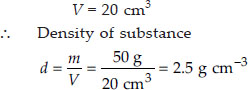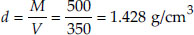Thrust and Pressure - NCERT Questions
Q 1.

Why is it difficult to hold a school bag having a strap made of a thin and strong string?

SOLUTION:

We know that pressure = force/area. When the string of the school bag is thin, its area is small and as such the pressure exerted on the shoulders or the hands supporting the bag is large. In this case, the force acting on the shoulders or the hand which support the bag is equal to the weight of the bag. In case, the strap is broad (as is usually the case), the area on which the force (weight of the bag) acts is large and the pressure is reduced (as the weight of the bag is now distributed over a larger area).

Q 2.

What do you mean by buoyancy?

SOLUTION:

Whenever an object is immersed in a liquid, either partially or fully, an upward force is exerted on the object by the liquid. This upward force is called upthrust or buoyant force or force of buoyancy and the property of the fluid due to which this upthrust is exerted on the object is called buoyancy.

Q 3.

Why does an object float or sink when placed on the surface of water?

SOLUTION:

When the object has density less than 1 g cm–3, then it floats on the surface of water, because, it always displaces more weight of water than its own weight. As buoyant force is more than its own weight, therefore, it floats. When the object has density more than 1 g cm–3, then it sinks in water, because it always displaces less weight of water than its own weight. As buoyant force is less than its own weight, therefore, it sinks.

Q 4.

You find your mass to be 42 kg on a weighing machine. Is your mass more or less than
42 kg?

SOLUTION:

In fact, a weighing machine is a sort of spring balance which measure the weight (and not the mass) of a body. When we stand on the weighing machine, our weight (which is due to gravitational attraction of the earth) acts vertically downwards. But the buoyancy due to air on our body acts vertically upwards. As a result of this, our apparent weight (true weight – buoyant force) is less than the true weight. Since the weighing machine measures the apparent weight, our true weight is more, i.e., more than 42 kg.

Q 5.

You have a bag of cotton and an iron bar, each indicating a mass of 100 kg when measured on a weighing machine. In reality, one is heavier than other. Can you say which one is heavier and why?

SOLUTION:

We know that true weight = apparent weight + upthrust. The cotton bag is heavier than the iron bar. This is due to the reason, that the bag of cotton which has more volume (as it has less density) than the iron bar (which has more density), experiences more upthrust due to air.

Q 6.

Why will a sheet of paper fall slower than one that is crumpled into a ball?

SOLUTION:

A sheet of paper will fall slower than the one that is crumpled into a ball. This is because the air offers resistance due to friction to the motion of the falling objects. The resistance offered by air to the sheet of paper is more than the resistance offered by air to the paper ball because the sheet has larger area.

Q 7.

In what direction does the buoyant force on an object immersed in a liquid act?

SOLUTION:

If an object is immersed in a liquid then the buoyant force due to liquid acts on the object in vertically upward direction.

Q 8.

Why does a block of plastic released under water come up to the surface of water?

SOLUTION:

The buoyant force acting on the block of plastic is more than its weight. As a result of this, it comes up when released under water. The cause of this larger buoyant force on the block of plastic is due to its density being lesser than that of water.

Q 9.

The volume of 50 g of a substance is 20 cm3. If the density of water is 1 g cm–3, will the substance float or sink?

SOLUTION:

Density of water
dw = 1 g cm–3 Mass of substance
m = 50 g
Volume of substance
V = 20 cm3
∴ Density of substanceAs the density of the substance is greater than that of water, the given substance will sink in water.

Q 10.

The volume of 500 g sealed packet is
350 cm3. Will the packet float or sink in water if the density of water is 1 g cm–3? What will be the mass of the water displaced by this packet?

SOLUTION:

Here, mass of packet, M = 500 g
volume of the packet, V = 350 cm3
Clearly, density of packet,Since the density of packet is more than density of water, the packet will sink.
As the packet is fully submerged in water,
mass of water displaced by the packet
= volume of the packet × density of water
= (350 cm3) × (1 g/cm3)
= 350 g